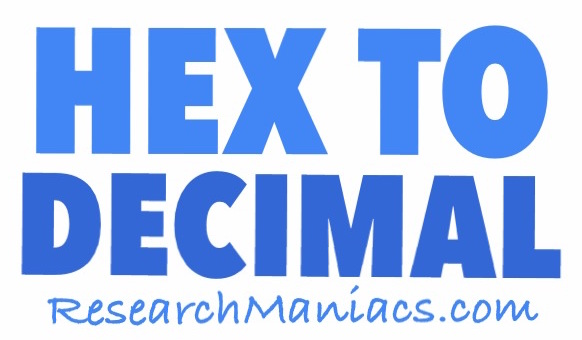Convert 1CD Hex to DecimalHere we will show you how to convert 1CD Hex to Decimal and then give you the answer.

Step 1) Count the digits in 1CD, which in this case is 3 digits, and convert each digit to Hex if necessary per Table 1.

Step 2) Starting from the last number in Step 1, multiply the first number with 1, the second number with 16, and the third number with 256. (See Table 2 for details.)

Step 3) Add up all the numbers you got in Step 2 to get the answer.

Following the instructions above, your math should look like this:

13 x 1 = 13
12 x 16 = 192
1 x 256 = 256

13 + 192 + 256 = 461

Thus, we got the following answer:

1CD = 461

TABLE 1:

If any digit in Step 1 is a letter, use the Hex to Decimal table below to convert the letter to a number.

 HEX A B C D E F = = = = = = Dec 10 11 12 13 14 15

TABLE 2:

Here are the numbers we used to multiply each digit in Step 2:

1st digit: 1
2nd digit: 16
3rd digit: 256
4th digit: 40,96
5th digit: 65,536
6th digit: 1,048,576
7th digit: 16,777,216

Convert 1CE Hex to Decimal
You just learned how to convert 1CD Hex to Decimal. Do you think you can convert HEX on your own now? If so, go here to try the next number we converted on our list.

1CD HEX Color
Do you want to see what the 1CD Hex color looks like? If so, check it out here!

Hex to Decimal Converter
On this page we converted the Hexadecimal number 1CD to Decimal. Go here to convert another Hex number.